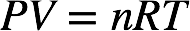# Problem: Cavendish prepared hydrogen in 1766 by the novel method of passing steam through a red-hot gun barrel:4 H2O (g) + 3 Fe(s) ⟶ Fe3O4 (s) + 4 H2 (g) (a) Outline the steps necessary to answer the following question: What volume of H2 at a pressure of 745 torr and a temperature of 20 °C can be prepared from the reaction of 15.0 g of H2O?

🤓 Based on our data, we think this question is relevant for Professor Selampinar's class at UCONN.

###### FREE Expert Solution

We can use the ideal gas law to determine the volume of H2 produced by the reaction.We first need to determine the moles of H2 produced from 15.0 g H2O. The molar mass of H2O is 2(1.01 g/mol H) + 16.00 g/mol O = 18.02 g/mol. From the given chemical equation, 4 moles of H2O form 4 moles of H2.###### Problem Details

Cavendish prepared hydrogen in 1766 by the novel method of passing steam through a red-hot gun barrel:

4 H2O (g) + 3 Fe(s) ⟶ Fe3O4 (s) + 4 H2 (g)

(a) Outline the steps necessary to answer the following question: What volume of H2 at a pressure of 745 torr and a temperature of 20 °C can be prepared from the reaction of 15.0 g of H2O?